Simple semi-group

(diff) ← Older revision | Latest revision (diff) | Newer revision → (diff)
A semi-group not containing proper ideals or congruences of some fixed type. Various kinds of simple semi-groups arise, depending on the type considered: ideal-simple semi-groups, not containing proper two-sided ideals (the term simple semi-group is often used for such semi-groups only); left (right) simple semi-groups, not containing proper left (right) ideals; (left, right)-simple semi-groups, semi-groups with a zero not containing proper non-zero two-sided (left, right) ideals and not being two-element semi-groups with zero multiplication; bi-simple semi-groups, consisting of one-class (cf. Green equivalence relations);-bi-simple semi-groups, consisting of two-classes one of which is the null class; and congruence-free semi-groups, not having congruences other than the universal relation and the equality relation.
Every left or right simple semi-group is bi-simple; every bi-simple semi-group is ideal-simple, but there are ideal-simple semi-groups that are not bi-simple (and even ones for which all the-classes consist of one element). The most important type of ideal-simple semi-groups (-simple semi-groups) are the completely-simple semi-groups (completely-simple semi-groups, cf. Completely-simple semi-group). The most important examples of bi-simple but not completely-simple semi-groups are: the bicyclic semi-groups and the four-spiral semi-group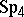(cf. Bicyclic semi-group; ). The latter,, is given by generators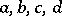and defining relations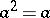,,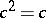,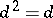,,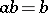,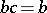,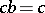,,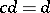,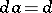. It is isomorphic to a Rees semi-group of matrix type over a bicyclic semi-group with generators, where, with sandwich-matrix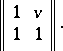In a sense,is minimal among the bi-simple not completely-simple semi-groups generated by a finite number of idempotents, and quite often it arises as a sub-semi-group of those semi-groups.
Right simple semi-groups are also called semi-groups with right division, or semi-groups with right invertibility. The reason for this terminology is the following equivalent property of such semi-groups: For any elementsthere is ansuch that. The right simple semi-groups containing idempotents are precisely the right groups (cf. Right group). An important example of a right simple semi-group without idempotents is given by the semi-groups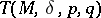of all transformationsof a setsuch that: 1) the kernel ofis the equivalence relationon; 2) the cardinality of the quotient set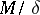is; 3) the set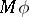intersects each-class in at most one element; and 4) the set of-classes disjoint fromhas infinite cardinality, and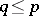. The semi-groupis called a Teissier semi-group of type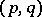, and, ifis the equality relation, it is called a Baer–Levi semi-group of type(cf. , ). A Teissier semi-group is an example of a right simple semi-group without idempotents that does not necessarily satisfy the right cancellation law. Every right simple semi-group without idempotents can be imbedded in a suitable Teissier semi-group, while every such semi-group with the right cancellation law can be imbedded in a suitable Baer–Levi semi-group (in both cases one can take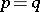).
Various types of simple semi-groups often arise as "blocks" from which one can construct the semi-groups under consideration. For classical examples of simple semi-groups see Completely-simple semi-group; Brandt semi-group; Right group; for bi-simple inverse semi-groups (including structure theorems under certain restrictions on the semi-lattice of idempotents) see , , . There are ideal-simple inverse semi-groups with an arbitrary number of-classes. In the study of imbedding of semi-groups in simple semi-groups one usually either indicates conditions for the possibility of the corresponding imbedding, or establishes that any semi-group can be imbedded in a semi-group of the type considered. E.g., any semi-group can be imbedded in a bi-simple semi-group with an identity (cf. ), in a bi-simple semi-group generated by idempotents (cf. ), and in a semi-group that is simple relative to congruences (which may have some property given in advance: the presence or absence of a zero, completeness, having an empty Frattini sub-semi-group, etc., cf. ).